Function Repository Resource:

# BitStringLinearSolve

A memory efficient form of solving linear systems modulo 2

Contributed by: Daniel Lichtblau
 ResourceFunction["BitStringLinearSolve"][{n1,n2,…},rhs,ncols]] solves the linear system {n1,n2,…}.x=rhs where {n1,n2,…} is a list of integers corresponding to bit vectors of length ncols, and rhs is an explicit vector of zeros and ones.

## Details and Options

The result is a 0‐1 vector corresponding to the solution one would obtain using LinearSolve with the option setting Modulus2 on the bit vectors that correspond to the matrix.
If no solution exists, ResourceFunction["BitStringLinearSolve"] will return an empty list.
ResourceFunction["BitStringLinearSolve"] returns one solution. Others may be found by adding multiples of null vectors mod 2 that may be computed using the resource function BitStringNullSpace.
The number of columns must be made explicit to account for the possibility of leading zeros in all rows.
ResourceFunction["BitStringLinearSolve"] is intended for dense linear algebra. Sparse methods might be better suited for linear algebra modulo 2 when most matrix entries are zeros.

## Examples

### Basic Examples (2)

Solve a simple example for a bit vector:

 In:=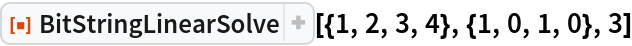Out=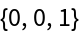Create a random matrix of bit vectors and a random right hand side:

 In:=Out=Solve the corresponding linear system modulo 2:

 In:=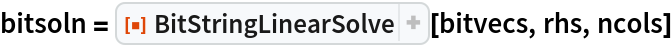Out=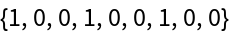### Scope (2)

Create a large array of bit strings to use as a matrix, along with a suitable right hand side vector:

 In:=BitStringLinearSolve is especially useful when working with matrices that might otherwise require excessive memory:

 In:=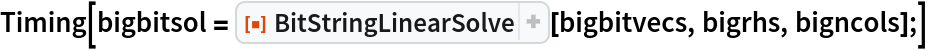Out=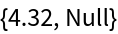### Properties and Relations (5)

Here is the matrix from which the bit vectors were created:

 In:=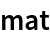Out=We see that the prior result agrees with LinearSolve:

 In:=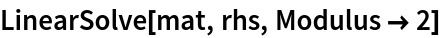Out=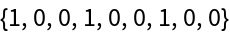In:=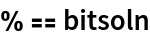Out=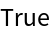Similarly, create a matrix of explicit 0‐1 vectors corresponding to the large integers:

 In:=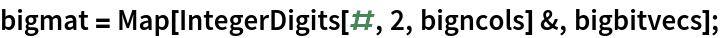LinearSolve is substantially slower as compared to BitStringLinearSolve:

 In:=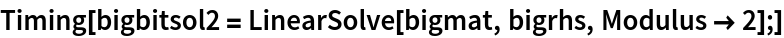Out=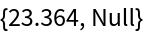Check that the results are the same:

 In:=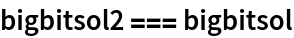Out=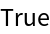## Requirements

Wolfram Language 11.3 (March 2018) or above

## Version History

• 1.0.0 – 03 April 2019